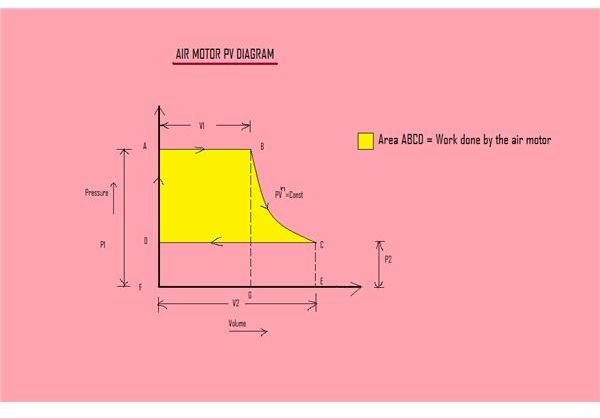# Air Motors – How to Compute Work Done per Air Cycle

## Introduction

There are lot of situations where we can not use electric motors. Sometimes the reason may be that the electric sparks produced might create a huge explosion or where electric cables may prove to be more dangerous. Air motors can prove to be much more satisfactory in situations like this.

Some of the advantages of air motors are:

• Air motors can be used in an atmosphere where even electric motors are not permitted
• For the same amount of power, the size of an air motor is very small when compared to an electric motor

• Air motors are very expensive

## Working of an air motor

The working of air motor is very similar to that of a reciprocating steam engine, except that the working medium here is air. In an air motor, compressed air from the air compressor or air reservoir enters into the cylinder of the air motor and pushes the piston downwards. The downward movement of the piston rotates the crank shaft. Work is done when the piston moves downward.

To understand the working of an air motor, let us see about a single cylinder reciprocating air motor without clearance.According to the PV diagram (click to enlarge), compressed air enters into the cylinder at point A with a pressure P1. Now the piston starts to move downwards. At point B, the air supply is cut-off. From this point the air inside the cylinder of the air motor starts to expand until point C. The air will be exhausted into the atmosphere at a constant pressure P2.

## Work done by the air per cycle

Let us assume the following:

P1 – Pressure of the compressed air entering into the cylinder of air motor at point A,

P2 – Pressure of the air at point D,

V1 – Volume of the compressed air entering into the cylinder of air motor at point A,

V2 – Volume of the compressed air at point D,

n – Polytropic index,

R – Universal gas constant,

T1 – Temperature of the air at point A.

The area of the indicator diagram gives the work done by the air motor.

Therefore the work done by the air per cycle is:

W = Area ABCD

= Area ABGF + Area BCEG – Area DCEF

= P1V1 + ((P1V1– P2V2)/ (n-1)) – P2V2

= (n P1V1 – P1V1 + P1V1 – n P2V2+ P2V2 – P2V2)/(n – 1)

= (n/ (n – 1)) ( P1V1– P2V2)

= (n/ (n – 1)) P1V1 (1 – (P2/P1) ((n – 1)/n))

W = (n/ (n – 1)) mRT1 (1 – (P2/P1) ((n – 1)/n))

(since PV = mRT)

Therefore the work done by the air per cycle is W = (n/ (n – 1)) mRT1 (1 – (P2/P1) ((n – 1)/n))